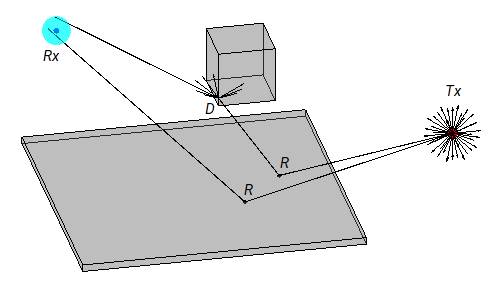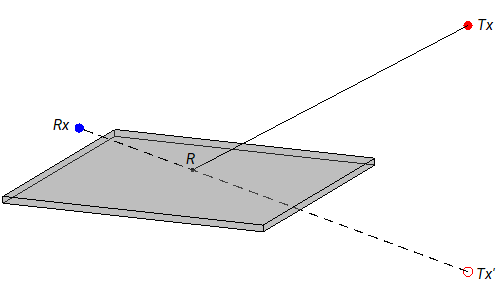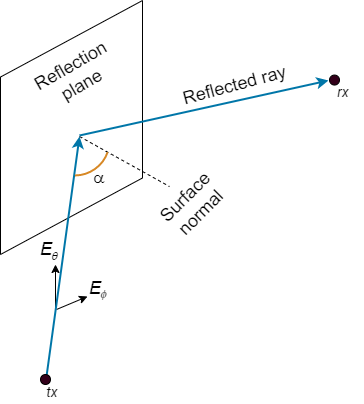## Ray Tracing for Wireless Communications

### Introduction

Wireless communication systems use radio waves to transmit signals. Propagation modeling enables you to estimate the strength of signals based on system parameters such as frequency, antenna height, terrain properties, and building properties.

Theoretical and empirical models estimate path loss based on range, and are valid only for those environments that resemble the modeling environment. As a result, these models usually do not provide accurate temporal or spatial information. Unlike these models, ray tracing models are specific to the 3-D environment, and are therefore appropriate for scenarios such as urban environments.

For propagation modeling, a ray is an individual radio signal that :

• Travels in a straight line through a homogeneous medium.

• Obeys the laws of reflection, refraction, and diffraction.

• Carries energy. Propagation models treat rays like tubes, where the energy density on the cross section becomes smaller as the ray interacts with the environment.

For a given 3-D environment, ray tracing models use numerical simulations to:

• Predict the paths of rays from transmitters to receivers. The models can find many rays from a transmitter to a receiver. The models derive the angle of departure, angle of arrival, and time of arrival from the paths.

• Estimate the path loss and phase change for each ray. Total path loss is the sum of interaction losses, free space loss, and, optionally, atmospheric loss.

A ray interacts with the environment in several ways .

InteractionDescription

Line-of-sight (LOS)

The ray travels directly from the transmitter to the receiver.

Reflection

The ray reflects off a surface according to the law of reflection.

Refraction (transmission)

The ray refracts as it moves into a new medium, according to the law of refraction.

Diffraction

The ray diffracts off a surface according to the law of diffraction. One ray can spawn many diffracted rays.

Scattering

The ray interacts with a rough surface such as the ocean or a building facade.

Use these functions to create ray tracing models, predict propagation paths, and calculate path losses and phase shifts.

• `propagationModel` — Create a ray tracing model as a `RayTracing` object. Specify options such as the ray tracing method, the maximum number of reflections, and surface materials. You can use ray tracing models as inputs when conducing RF analysis, such as when generating coverage maps by using the `coverage` function or when calculating total received power by using the `sigstrength` function.

• `raytrace` — Display propagation paths (rays) on a map or return propagation paths as `comm.Ray` objects. Each object represents the full path from the transmitter to the receiver, and contains information such as the path loss, phase shift, and types of surface interactions.

• `raypl` — Calculate the path loss and phase shift for a propagation path based on surface materials and antenna polarization types.

For examples that show ray tracing in indoor and urban environments, see Indoor MIMO-OFDM Communication Link using Ray Tracing and Urban Link and Coverage Analysis Using Ray Tracing, respectively.

### Ray Tracing Methods

The `propagationModel` and `raytrace` functions use a ray tracing model that finds LOS and non-line-of-sight (NLOS) paths.

• The model finds LOS paths by shooting a ray from the transmitter toward the receiver. If the ray does not interact with a surface before reaching the receiver, then an LOS path exists.

• The model finds NLOS paths by using either the shooting and bouncing rays (SBR) method  or the image method. You can specify the method by using the `propagationModel` function.

Choose a method based on the types of interactions you want to model, the computation speed, and the accuracy.

MethodInteraction TypesComputation SpeedComputation Accuracy

SBR

Includes effects from reflection and does not include effects from diffraction, refraction, or scattering.

Supports calculation of approximate propagation paths for up to ten path reflections.

Computational complexity increases linearly with the number of reflections. As a result, the SBR method is generally faster than the image method.

The locations of receiver sites calculated by the SBR method are not exact. The accuracy of the calculated propagation paths decreases as the length of the paths increases.

Image

Includes effects from reflection and does not include effects from diffraction, refraction, or scattering.

Supports up to two path reflections.

Computational complexity increases exponentially with the number of reflections.

Calculates exact propagation paths.

#### SBR Method

This figure illustrates the SBR method for calculating propagation paths from a transmitter, Tx, to a receiver, Rx.The SBR method launches many rays from a geodesic sphere centered at Tx. The geodesic sphere enables the model to launch rays that are approximately uniformly spaced.

Then, the method traces every ray from Tx and can model different types of interactions between the rays and surrounding objects, such as reflections, diffractions, refractions, and scattering. Note that the implementation considers only reflections.

• When a ray hits a flat surface, shown as R, the ray reflects based on the law of reflection.

• When a ray hits an edge, shown as D, the ray spawns many diffracted rays based on the law of diffraction . Each diffracted ray has the same angle with the diffracting edge as the incident ray. The diffraction point then becomes a new launching point and the SBR method traces the diffracted rays in the same way as the rays launched from Tx. A continuum of diffracted rays forms a cone around the diffracting edge, which is commonly known as a Keller cone . The current implementation of the SBR method does not consider diffraction.

For each launched ray, the method surrounds Rx with a sphere, called a reception sphere, with a radius that is proportional to the angular separation of the launched rays and the distance the ray travels. If the ray intersects the sphere, then the model considers the ray a valid path from Tx to Rx.

#### Image Method

This figure illustrates the image method for calculating the propagation path of a single reflection ray for the same transmitter and receiver as the SBR method. The image method locates the image of Tx with respect to a planar reflection surface, Tx'. Then, the method connects Tx' and Rx with a line segment. If the line segment intersects the planar reflection surface, shown as R in the figure, then a valid path from Tx to Rx exists. The method determines paths with multiple reflections by recursively extending these steps.### Propagation Loss

The ray tracing model used by the `propagationModel`, `raytrace`, and `raypl` functions calculates reflection losses by tracking the horizontal and vertical polarizations of signals through the propagation path. Total power loss is the sum of free space loss, and reflection loss.

#### Effect of Surface Materials

When a ray interacts with a surface, the surface material impacts the reflection losses.

The ray tracing model incorporates building and surface materials into the propagation loss calculations by using the complex relative permittivity of the surface, εr. The ITU-R P.2040-1  and ITU-R P.527  recommendations include methods, equations, and values used to calculate εr for a range of frequencies.

The equations for εr are:

${\epsilon }_{r}={\epsilon }_{r}{}^{\prime }+j{\epsilon }_{r}{}^{\prime \text{​}\prime }$

${\epsilon }_{r}{}^{\prime \text{​}\prime }=\frac{\sigma }{2\pi {\epsilon }_{0}f}$,

where:

• εr' is the real relative permittivity.

• σ is the conductivity in S/m.

• ε0 is the permittivity of free space (electric constant).

• f is the frequency in Hz.

For building materials, the ray tracing model calculates εr' and σ as:

${\epsilon }_{r}{}^{\prime }=a{f}^{b}$

$\sigma =c{f}^{d}$,

where a, b, c, and d are constants determined by the surface material. For readability, this table shows the frequency range in GHz.

Material ClassReal Part of Relative PermittivityConductivity (S/m)Frequency Range (GHz)
abcd

Vacuum (~ air)

1

0

0

0

[0.001, 100]

Concrete

5.31

0

0.0326

0.8095

[1, 100]

Brick

3.75

0

0.038

0

[1, 10]

Plasterboard

2.94

0

0.0116

0.7076

[1, 100]

Wood

1.99

0

0.0047

1.0718

[0.001, 100]

Glass

6.27

0

0.0043

1.1925

[0.1, 100]

Ceiling board

1.50

0

0.0005

1.1634

[1, 100]

Chipboard

2.58

0

0.0217

0.78

[1, 100]

Floorboard

3.66

0

0.0044

1.3515

[50, 100]

Metal

1

0

107

0

[1, 100]

Very dry ground

3

0

0.00015

2.52

[1, 10] only(a)

Medium dry ground

15

– 0.1

0.035

1.63

[1, 10] only(a)

Wet ground

30

– 0.4

0.15

1.30

[1, 10] only(a)

Note (a): For the three ground types (very dry, medium dry, and wet), the noted frequency limits cannot be exceeded.

For earth surfaces such as water, sea water, dry or wet ice, dry or wet soil, and vegetation, the ray tracing model calculates εr using the methods and equations presented in ITU-R P.527 .

#### Reflection Loss

The ray tracing model computes reflection loss by using the reflection matrix computations described in IEEE document 802.11-09/0334r8 .

This image shows a reflection path from a transmitter site Tx to a receiver site Rx.For a first-order signal reflection, the equation for reflection path loss, PLR, is:

$P{L}_{R}=J{V}_{rx}{}^{\prime }{H}_{ref1}J{V}_{tx}$,

where:

• JVrx and JVtx are 2-by-1 polarization vectors for the receiver and transmitter, specified as Jones vectors.

• Href1 is a reflection matrix.

The equation for the reflection matrix Href1 is:

${H}_{ref1}=\left(\begin{array}{cc}\mathrm{cos}\left({\Psi }_{rx}\right)& \mathrm{sin}\left({\Psi }_{rx}\right)\\ -\mathrm{sin}\left({\Psi }_{rx}\right)& \mathrm{cos}\left({\Psi }_{rx}\right)\end{array}\right)×\left(\begin{array}{cc}{R}_{\perp }\left(\alpha \right)& 0\\ 0& {R}_{\parallel }\left(\alpha \right)\end{array}\right)×\left(\begin{array}{cc}\mathrm{cos}\left({\Psi }_{tx}\right)& \mathrm{sin}\left({\Psi }_{tx}\right)\\ -\mathrm{sin}\left({\Psi }_{tx}\right)& \mathrm{cos}\left({\Psi }_{tx}\right)\end{array}\right)$,

where:

• The third and first terms are geometric coupling matrices. The third term recalculates the polarization vector from the basis of the transmitter coordinates to the basis of the incident plane. The first term recalculates the polarization vector from the basis of the incident plane to the basis of the receiver coordinates. Ψrx and Ψtx are the angles between the vertical electromagnetic field vector Eθ and a normal to the incident plane, n, at the receiver and transmitter, respectively.

• The second term is a polarization matrix, where R and R are the reflection coefficients for the horizontal and vertical polarizations, respectively.

The model accounts for the geometric coupling between horizontal and vertical polarizations only when both the transmitter and receiver antennas are polarized.

Ray tracing models calculate R and R by using the Fresnel equation:

${R}_{\parallel }\left(\alpha \right)=\frac{\mathrm{cos}\left(\alpha \right)-\sqrt{{\epsilon }_{r}-{\mathrm{sin}}^{2}\left(\alpha \right)}}{\mathrm{cos}\left(\alpha \right)+\sqrt{{\epsilon }_{r}-{\mathrm{sin}}^{2}\left(\alpha \right)}}$

${R}_{\perp }\left(\alpha \right)=\frac{\mathrm{cos}\left(\alpha \right)-\sqrt{\left({\epsilon }_{r}-{\mathrm{sin}}^{2}\left(\alpha \right)\right)/{\epsilon }_{r}{}^{2}}}{\mathrm{cos}\left(\alpha \right)+\sqrt{\left({\epsilon }_{r}-{\mathrm{sin}}^{2}\left(\alpha \right)\right)/{\epsilon }_{r}{}^{2}}}$,

where:

• α is the incident angle of the propagation vector.

• εr is the complex relative permittivity of the material.

The model computes higher-order reflections by using an additional geometric coupling matrix and polarization matrix for each reflection.

 Yun, Zhengqing, and Magdy F. Iskander. “Ray Tracing for Radio Propagation Modeling: Principles and Applications.” IEEE Access 3 (2015): 1089–1100. https://doi.org/10.1109/ACCESS.2015.2453991.

 Schaubach, K.R., N.J. Davis, and T.S. Rappaport. “A Ray Tracing Method for Predicting Path Loss and Delay Spread in Microcellular Environments.” In [1992 Proceedings] Vehicular Technology Society 42nd VTS Conference - Frontiers of Technology, 932–35. Denver, CO, USA: IEEE, 1992. https://doi.org/10.1109/VETEC.1992.245274.

 International Telecommunications Union Radiocommunication Sector. Propagation by diffraction. Recommendation P.526-15. ITU-R, approved October 21, 2019. https://www.itu.int/rec/R-REC-P.526-15-201910-I/en.

 Keller, Joseph B. “Geometrical Theory of Diffraction.” Journal of the Optical Society of America 52, no. 2 (February 1, 1962): 116. https://doi.org/10.1364/JOSA.52.000116.

 International Telecommunications Union Radiocommunication Sector. Effects of building materials and structures on radiowave propagation above about 100MHz. Recommendation P.2040-1. ITU-R, approved July 29, 2015. https://www.itu.int/rec/R-REC-P.2040-1-201507-I/en.

 International Telecommunications Union Radiocommunication Sector. Electrical characteristics of the surface of the Earth. Recommendation P.527-5. ITU-R, approved August 14, 2019. https://www.itu.int/rec/R-REC-P.527-5-201908-I/en.

 Maltsev, A., et al. "Channel models for 60 GHz WLAN systems." IEEE Document 802.11-09/0334r8, May 2010.

 McNamara, D. A., C. W. I. Pistorius, and J. A. G. Malherbe. Introduction to the Uniform Geometrical Theory of Diffraction. Boston: Artech House, 1990.